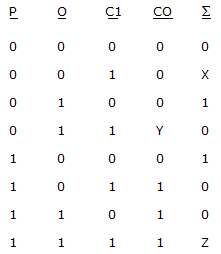# Digital Electronics - Digital Arithmetic Operations and Circuits

### Exercise :: Digital Arithmetic Operations and Circuits - General Questions

26.

One way to make a four-bit adder perform subtraction is by:

 A. inverting the output. B. inverting the carry-in. C. inverting the B inputs. D. grounding the B inputs.

Answer: Option C

Explanation:

No answer description available for this question. Let us discuss.

27.

What is the most important operation in binary-coded decimal (BCD) arithmetic?

 A. addition B. subtraction C. multiplication D. division

Answer: Option A

Explanation:

No answer description available for this question. Let us discuss.

28.

The range of positive numbers when using an eight-bit two's-complement system is:

 A. 0 to 64 B. 0 to 100 C. 0 to 127 D. 0 to 256

Answer: Option C

Explanation:

No answer description available for this question. Let us discuss.

29.

What are the two types of basic adder circuits?

 A. sum and carry B. half-adder and full-adder C. asynchronous and synchronous D. one- and two's-complement

Answer: Option B

Explanation:

No answer description available for this question. Let us discuss.

30.

The truth table for a full adder is shown below. What are the values of X, Y, and Z?A. X = 0, Y = 1, Z = 1 B. X = 1, Y = 1, Z = 1 C. X = 1, Y = 0, Z = 1 D. X = 0, Y = 0, Z = 1

Answer: Option B

Explanation:

No answer description available for this question. Let us discuss.

#### Current Affairs 2021

Interview Questions and Answers